# python验证码识别教程之灰度处理、二值化、降噪与tesserocr识别

1. 图像类
2. 滑动类
3. 点击类
4. 语音类

1. 灰度处理
2. 增加对比度(可选)
3. 二值化
4. 降噪
5. 倾斜校正分割字符
6. 建立训练库
7. 识别

```from claptcha import Claptcha

c = Claptcha("8069","/usr/share/fonts/truetype/freefont/FreeMono.ttf")
t,_ = c.write('1.png')``````apt-get install tesseract-ocr libtesseract-dev libleptonica-dev
pip install tesserocr```

```from PIL import Image
import tesserocr

p1 = Image.open('1.png')
tesserocr.image_to_text(p1)

'8069\n\n'```

```c = Claptcha("8069","/usr/share/fonts/truetype/freefont/FreeMono.ttf",noise=0.4)
t,_ = c.write('2.png')```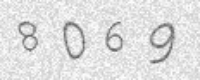```p2 = Image.open('2.png')
tesserocr.image_to_text(p2)
'8069\n\n'```

```c2 = Claptcha("A4oO0zZ2","/usr/share/fonts/truetype/freefont/FreeMono.ttf")
t,_ = c2.write('3.png')```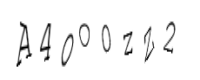```p3 = Image.open('3.png')
tesserocr.image_to_text(p3)
'AMOOZW\n\n'```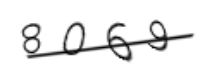```p4 = Image.open('4.png')
tesserocr.image_to_text(p4)
''```

```def binarizing(img,threshold):
"""传入image对象进行灰度、二值处理"""
img = img.convert("L") # 转灰度
w, h = img.size
# 遍历所有像素，大于阈值的为黑色
for y in range(h):
for x in range(w):
if pixdata[x, y] < threshold:
pixdata[x, y] = 0
else:
pixdata[x, y] = 255
return img```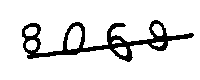```def depoint(img):
"""传入二值化后的图片进行降噪"""
w,h = img.size
for y in range(1,h-1):
for x in range(1,w-1):
count = 0
if pixdata[x,y-1] > 245:#上
count = count + 1
if pixdata[x,y+1] > 245:#下
count = count + 1
if pixdata[x-1,y] > 245:#左
count = count + 1
if pixdata[x+1,y] > 245:#右
count = count + 1
if pixdata[x-1,y-1] > 245:#左上
count = count + 1
if pixdata[x-1,y+1] > 245:#左下
count = count + 1
if pixdata[x+1,y-1] > 245:#右上
count = count + 1
if pixdata[x+1,y+1] > 245:#右下
count = count + 1
if count > 4:
pixdata[x,y] = 255
return img```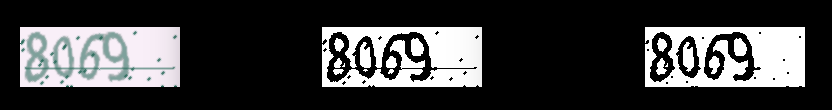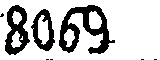```p7 = Image.open('7.png')
tesserocr.image_to_text(p7)
'8069 ,,\n\n'```